Learn data science and machine learning by building real-world projects on Jovian

2019 Indian General Elections Data AnalysisThis project is to perform Exploratory Data Analyis on the 2019 Indian General Elections dataset. Here we use various Python libraries to perform Data Cleaning and Visualization. The Dataset which is used in this project is from Kaggle, authored by the user Prakrut Chauhan.

• Link to the Dataset used - Source
The dataset contains information of all the candidates who contested the elections from various Constituencies. Data includes personal information like Assets, Education, Criminal Record, etc. as well as electoral information such as Contesting Constituency, Political Party, Total Votes received, etc.

The Libraries used in the Project are:

To install all required libraries, run the following Command:

pip install matplotlib seaborn numpy pandas jovian --upgrade

The following Tasks are implemented in the Project:

LET'S DIVE INTO THE PROJECT !!!

The dataset is unpacked and opened using the opendatasets package from the jovian library.

!pip install jovian opendatasets --upgrade --quiet
dataset_url = 'https://www.kaggle.com/prakrutchauhan/indian-candidates-for-general-election-2019'

import opendatasets as od

data_dir = './indian-candidates-for-general-election-2019'
import os
os.listdir(data_dir)
['LS_2.0.csv']

Let us save and upload our work to Jovian before continuing.

project_name = "general-elections-analysis"
import jovian
jovian.commit(project=project_name)
[jovian] Updating notebook "ash007online/general-elections-analysis" on https://jovian.ai [jovian] Committed successfully! https://jovian.ai/ash007online/general-elections-analysis

The raw data is now obtained. First we need to clean and simplify the data in order to prepare it for Analysis.

Data Preparation and Cleaning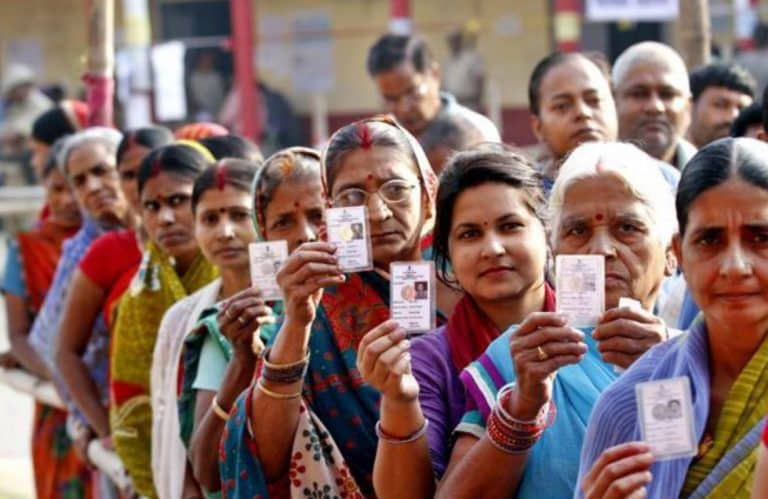The .csv file which we downloaded from Kaggle is now converted to a Pandas DataFrame and cleaned to extract only the columns which will be needed for analysis.
Holes and anomalies in the data (which were found in due course of Analysis) have also been rectified here itself to avoid loopholes later on.

import pandas as pd

Here we first load the dataset onto a DataFrame.

raw_election_data

The function convert(x) below is used to convert the ASSETS and LIABILITIES columns of the raw_election_data DataFrame into numeric values.

def convert(x):
'''
Extract the numeric value from the passed string and return it as float
'''
if str(x) == 'R':
#         this is to ensure only valid values (and not NaN values) are converted
return float(str(x).split().replace(",", ""))
return 0.0
# default 0
raw_election_data.ASSETS = raw_election_data.ASSETS.apply(convert)
raw_election_data.LIABILITIES = raw_election_data.LIABILITIES.apply(convert)
# convert the ASSETS and LIABILITIES to numeric data

# the above can also be done using lambda function
raw_election_data.sample(5)
# check if the applied operations were successful

When the data was analysed later, it was found that the following categories in EDUCATION column would cause some uncertainities in the visualization process. Hence those are updated here itself, for all subsequent DataFrames.

These are holes in the data which must be fixed beforehand to avoid errors later.

raw_election_data.at[192, "WINNER"] = 1
raw_election_data.at[702, "WINNER"] = 1
raw_election_data.at[951, "WINNER"] = 1
raw_election_data.at[1132, "WINNER"] = 1
raw_election_data.at[172, "WINNER"] = 0

Now we drop the unnecessary columns and create a new DataFrame candidates_df and change some column names for visualization purposes.

raw_election_data.columns
Index(['STATE', 'CONSTITUENCY', 'NAME', 'WINNER', 'PARTY', 'SYMBOL', 'GENDER',
'CRIMINAL\nCASES', 'AGE', 'CATEGORY', 'EDUCATION', 'ASSETS',
'OVER TOTAL ELECTORS \nIN CONSTITUENCY',
'OVER TOTAL VOTES POLLED \nIN CONSTITUENCY', 'TOTAL ELECTORS'],
dtype='object')
'OVER TOTAL ELECTORS \nIN CONSTITUENCY', 'OVER TOTAL VOTES POLLED \nIN CONSTITUENCY'], axis=1)
# take out the unnecessary columns
candidates_df.sort_values(["STATE", "CONSTITUENCY"], inplace = True)
# rename some of the columns and sort the data with respect to State and Constituency columns
candidates_df.info()
<class 'pandas.core.frame.DataFrame'> Int64Index: 2263 entries, 105 to 2171 Data columns (total 14 columns): # Column Non-Null Count Dtype --- ------ -------------- ----- 0 STATE 2263 non-null object 1 CONSTITUENCY 2263 non-null object 2 NAME 2263 non-null object 3 WINNER 2263 non-null int64 4 PARTY 2263 non-null object 5 GENDER 2018 non-null object 6 CRIMINAL CASES 2018 non-null object 7 AGE 2018 non-null float64 8 CATEGORY 2018 non-null object 9 EDUCATION 2018 non-null object 10 ASSETS 2263 non-null float64 11 LIABILITIES 2263 non-null float64 12 TOTAL VOTES 2263 non-null int64 13 TOTAL ELECTORS 2263 non-null int64 dtypes: float64(3), int64(3), object(8) memory usage: 265.2+ KB

Converting the data of CRIMINAL CASES column to numeric type.

candidates_df["CRIMINAL CASES"] = pd.to_numeric(candidates_df["CRIMINAL CASES"], errors = 'coerce').convert_dtypes()
candidates_df

Some more editing is done, and only the personal details of non-NOTA candidates is extracted and stored in a new DataFrame candidates_personal_df.

candidates_personal_df = candidates_df[candidates_df.NAME != "NOTA"]
candidates_personal_df = candidates_personal_df.drop(["TOTAL VOTES", "TOTAL ELECTORS"], axis = 1)
candidates_personal_df
candidates_personal_df.describe()
# works on only numeric data

Another DataFrame winners_df is created which contains the details of only the winning candidates.
Some operations are performed to shape the DataFrame as required.

winners_df = candidates_df[candidates_df.WINNER == 1].sort_values(["STATE", "CONSTITUENCY"]).reset_index()
# extract the list of winners
winners_df.drop(["index", "WINNER"], axis = 1, inplace = True)
winners_df
print("Number of Parties which fielded at least 1 candidate: ", candidates_df.PARTY.unique().shape-2)
# -2 : 1 for independent candidates and 1 for NOTA
Number of Parties which fielded at least 1 candidate: 131
print("Number of Independent Candidates who contested the elections: ", candidates_df[candidates_df.PARTY == 'IND'].shape)
Number of Independent Candidates who contested the elections: 201
print("Number of Parties which won at least 1 seat: ", winners_df.PARTY.unique().shape - 1)
# -1 : for independent winners
Number of Parties which won at least 1 seat: 35
print("Number of Independent Winners: ", winners_df[winners_df.PARTY == 'IND'].shape)
Number of Independent Winners: 4

Exploratory Analysis and VisualizationIn this part we analyse the simplified Dataset to extract some basic information and trends about the outcome of the elections and the candidates.

Let's begin by importingmatplotlib.pyplot and seaborn.

import seaborn as sns
import matplotlib
import matplotlib.pyplot as plt
%matplotlib inline

sns.set_style('darkgrid')
matplotlib.rcParams['font.size'] = 14
matplotlib.rcParams['figure.figsize'] = (15, 10)
matplotlib.rcParams['figure.facecolor'] = '#00000000'
import numpy as np

Seat Share

Here we calculate how many seats were won by each party, and the percentage seat distribution of the House.

all_party_seats = winners_df.PARTY.value_counts().sort_values(ascending = False)
# frequency of each PARTY in the winner list
all_party_seats
BJP       303
INC        52
DMK        23
YSRCP      22
AITC       22
SHS        18
JD(U)      16
BJD        12
BSP        10
TRS         9
LJP         6
NCP         5
SP          5
CPI(M)      5
IND         4
IUML        3
JKN         3
TDP         3
AIMIM       2
SKM         1
AJSUP       1
JMM         1
VCK         1
AAP         1
RLTP        1
KEC(M)      1
JD(S)       1
NDPP        1
MNF         1
RSP         1
NPF         1
AIUDF       1
NPEP        1
Name: PARTY, dtype: int64
others = all_party_seats[all_party_seats<10].sum()
# simplifying the output for visualization purposes
seat_distribution = all_party_seats[all_party_seats>=10].append(pd.Series({"Others":others}))
seat_distribution
BJP       303
INC        52
DMK        23
YSRCP      22
AITC       22
SHS        18
JD(U)      16
BJD        12
BSP        10
Others     64
dtype: int64

Plot the distribution as a pie chart.

plt.figure(figsize = (10,8))
plt.title("SEAT SHARE")
# basic details

plt.pie(seat_distribution, labels = seat_distribution.index,
colors = ['#f97d09', '#00bdfe', '#dc143c', '#0266b4', '#24b44c', '#ff6634',
'#203354', '#105e27', '#22409a', '#FFFFFF'],
wedgeprops = {'edgecolor' : 'black', 'linewidth' : 0.75, 'antialiased' : True})
# pie chart created using the Data, labels, colors, and wedge border properties
# colors are customised according to each party's colors

seat_percent = round((seat_distribution/seat_distribution.sum())*100,2)
legend = seat_percent.index + " (" + seat_percent.values.astype(str) + "%) - " + seat_distribution.values.astype(str)
# the legend would the percentage seat share of each party (& others)

plt.legend(legend, loc = "right", bbox_to_anchor = (1.6,0.5));
# legend is placed outside the main chart accordingly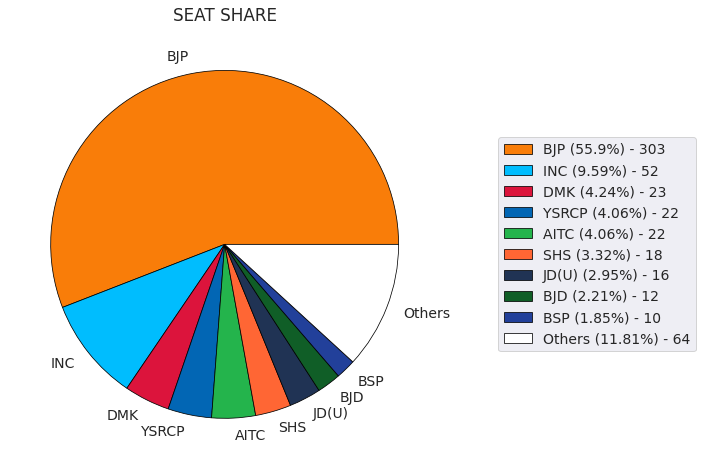As we can see, the BJP was the single largest party with more than 50% of the seats in the House, with INC at a distant second.
Other regional parties like DMK, YSRCP, AITC, BJD won some seats in their respective states, but no Alliance could pose as an alternative to BJP.

Age

In this part we analyse the number of candidates, both contestants and winners, in each age group.

We plot a nested histogram with each bin of size 5,
And calculate the mean, maximum and minimum age of all candidates and winners.

plt.figure(figsize = (20,10))
plt.title("Age of Candidates Contested and Won", fontsize=20)
plt.xlabel("Age", fontsize=17)
plt.ylabel("Number of candidates", fontsize=17)
# put the basic labelling

plt.xticks(fontsize=15)
plt.yticks(fontsize=15)
# axes ticks size

sns.histplot(data = candidates_personal_df, x = 'AGE', bins = np.arange(20,100,5), color = 'indigo', alpha = 0.5)
sns.histplot(data = winners_df, x = 'AGE', bins = np.arange(20,100,5), color = 'lightgreen', alpha = 1)
# two histograms plotted, Won over Contested to show the relative percentage

plt.legend(["Candidates Contested", "Candidates Won"], fontsize = 15)
# legend to the plot

plt.text(84.5, 238, "All Candidates:")
plt.figtext(0.77, 0.63, round(candidates_personal_df.describe().AGE[['mean', 'min', 'max']], 2).to_string())

plt.text(84.5,185, "Winning Candidates:")
plt.figtext(0.77, 0.5, round(winners_df.describe().AGE[['mean', 'min', 'max']], 2).to_string());
# basic stats printed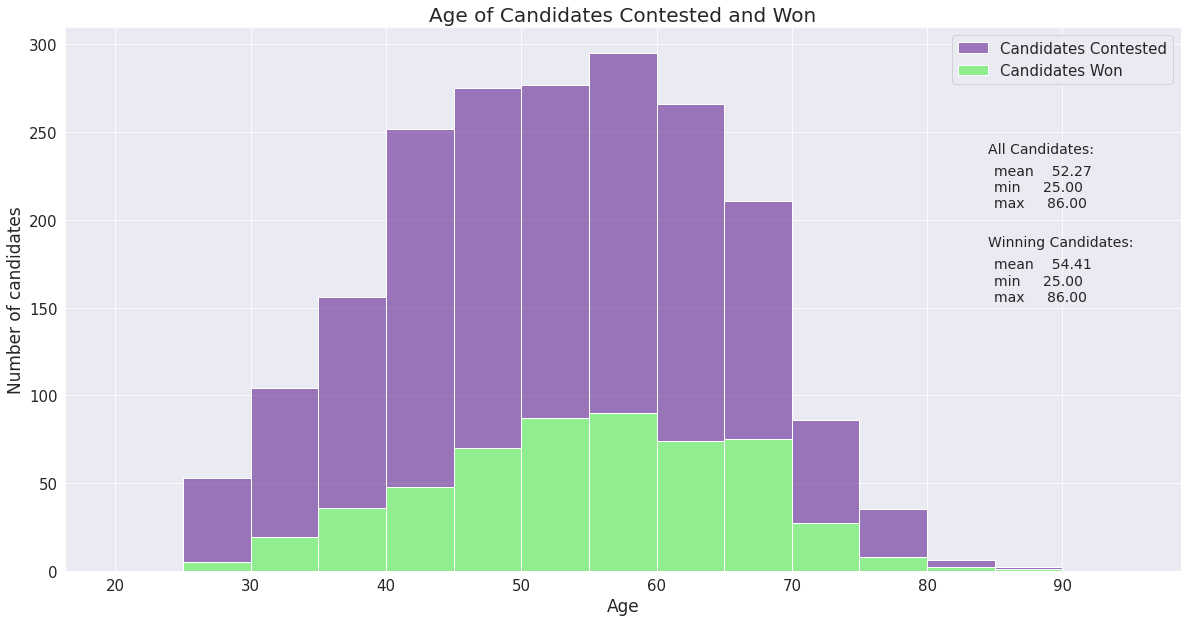As we can see from the Nested Histogram, the age group 55-60 has the maximum number of Candidates, and Winners, followed closely by the age group 50-55.
The average age of the house - 54 years also lies in this range. A majority of the winners are between the ages 45-70, which can be considered as the normal peak years of a Politician.

print("Youngest Member of the House:")
winners_df[(winners_df.AGE == 25)][["NAME", "PARTY", "STATE", "CONSTITUENCY"]].reset_index(drop = True)
Youngest Member of the House:Chandrani Murmu
print("Oldest Member of the House:")
winners_df[(winners_df.AGE == 86)][["NAME", "PARTY", "STATE", "CONSTITUENCY"]].reset_index(drop = True)
Oldest Member of the House:

Seat Category

Here we calculate the ratio of seats which have a special reservation status for candidates of different backward classes.

seat_category = winners_df.CATEGORY.value_counts()
# winners_df has 1 constituency only 1 time, so analysing its CATEGORY column will give the correct result
pd.DataFrame(seat_category)

Plot the distribution as a Pie chart:

sns.set_palette(sns.color_palette('Set2'))
plt.figure(figsize=(8,5))
plt.title("Distribution of Seats by Category", size=18, x = 0.52, y =0.95)

plt.pie(seat_category, labels = seat_category.index, autopct = '%1.1f%%', startangle = 45);
# percentage of seats shown on the plot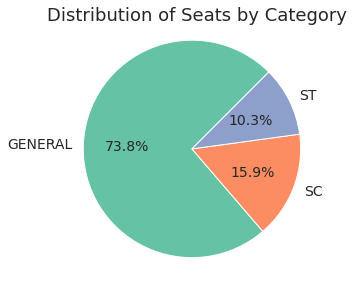As we can see, about 26% seats in the Lok Sabha are reserved for SC and ST candidates, which is appropriate as they comprise about 25% of the population (as per the 2011 Census).

Gender

In this section we see the gender diversity of the contesting candidates, as well as the winning Members of Parliament.

Plot the data as a horizontal bar chart.

gender_group = candidates_personal_df.groupby(["GENDER", "WINNER"]).size()
gender_group = gender_group.unstack()
gender_group = gender_group[[1,0]]
# a2a from stack overflow

# gender with winning condition is extracted as a dataframe

sns.set_palette(sns.color_palette("icefire"))
# color palette set
gender_group.plot(kind = 'barh', figsize = (15,6), title = "Gender Comparison of Contesting and Winning Candidates")
# horizontal bar plot created with Pandas

plt.legend(["Won", "Lost"])
plt.xlabel("Number of Candidates")
plt.ylabel("Gender")
# legend and labels set

plt.figtext(0.738,0.53, "Contesting Candidates:\n" +
round((candidates_personal_df.GENDER.value_counts(normalize=True)*100),2).to_string().replace("\n", "%\n")+"%")

plt.figtext(0.738,0.33, "Winning Candidates:\n" +
round((winners_df.GENDER.value_counts(normalize=True)*100),2).to_string().replace("\n", "%\n")+"%")

# Total candidates statistics (percentages) printed on the chart, with some applied String formatting to give the look

win_percent = round((winners_df.GENDER.value_counts()/candidates_personal_df.GENDER.value_counts())*100,2)
plt.figtext(0.395, 0.63, str(round(win_percent.MALE,2)) + "% candidates won")
plt.figtext(0.175, 0.25, str(round(win_percent.FEMALE,2))+ "% candidates won");
# percentage of winning, gender-wise printed on the chart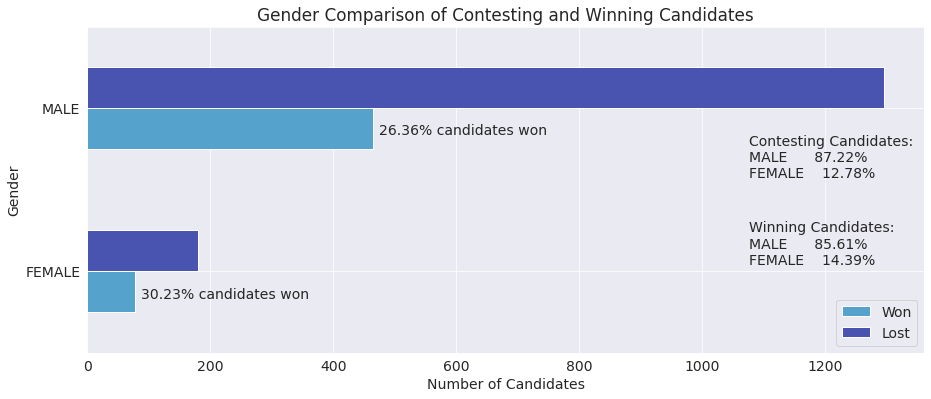print("No. of male MPs: ", winners_df.GENDER.value_counts()["MALE"])
print("No. of female MPs: ", winners_df.GENDER.value_counts()["FEMALE"])
No. of male MPs: 464 No. of female MPs: 78

As we can see, the House has 14.4% Female members and 85.6% Male members.

One surprising inference we can draw from the Analysis is that despite a higher percentage of Male Candidates contesting the elections than Female (87.2% vs 12.8%), the percentage of Female Contestants who won was greater than that of Male Contestants (30.2% vs 26.4%).
This means, a Female Candidate had a greater chance of winning the election than a Male Candidate.

Educational Qualifications

Here we analyse the educational qualifications of all the Winning Candidates.

winners_df["EDUCATION"].unique()
'Post Graduate', '12th Pass', '10th Pass', 'Others', '5th Pass',
'Illiterate', 'Literate'], dtype=object)
education = winners_df.EDUCATION.value_counts()
education = education.reindex(["Illiterate", "Literate", "5th Pass", "8th Pass", "10th Pass", "12th Pass", "Graduate",
# arrange the Series in a systematic order
education
Illiterate                  1
Literate                    1
5th Pass                    4
8th Pass                   12
10th Pass                  45
12th Pass                  69
Doctorate                  24
Others                     17
Name: EDUCATION, dtype: int64

Plot the data as a Bar Chart.

plt.figure(figsize=(15,7))
plt.xticks(rotation = 60);
# plot detailing

plt.xlabel("Education Status", fontsize = 15)
plt.ylabel("No. of Candidates", fontsize = 15)
plt.title("EDUCATIONAL QUALIFICATIONS OF WINNERS",fontsize = 18)
# labels and title

sns.barplot(x = education.index, y = education.values);
# plotting the barplot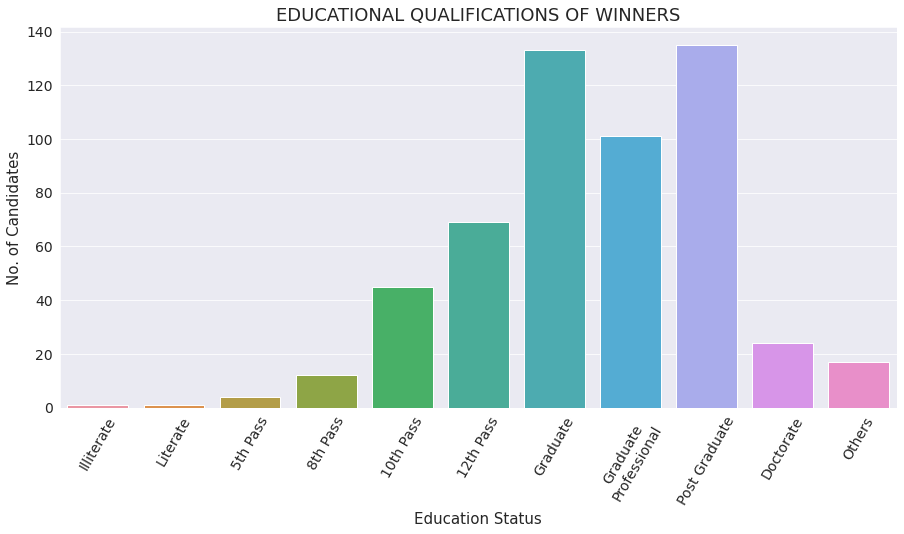We see, contrary to popular belief, most MPs are well educated and have at least a Graduate degree.
There are less than 150 MPs who are 12th Pass or below.

Here we selected some specific columns of the DataFrames and performed Analysis and Visualization on those data. Now we shall move onto more complex Analysis and answering specific Questions.Victorious Prime Minister Narendra Modi alongside BJP Party President Amit Shah

Now we shall pose some general Election related questions, and find the answers to those using Data Analysis, and Visualize them wherever possible.

Q1: Which States/UTs and Constituencies had the highest and the lowest Voter Turnout?Election Officers

We first create a new DataFrame votes_df with the sum of TOTAL VOTES column of the candidates_df DataFrame and the sum of TOTAL ELECTORS column of the winners_df DataFrame, grouping them by the STATE and CONSTITUENCY columns and append the VOTER TURNOUT in each constituency, as a column, to the end of the DataFrame.